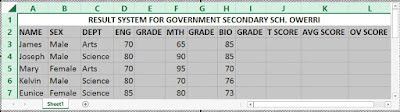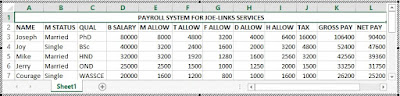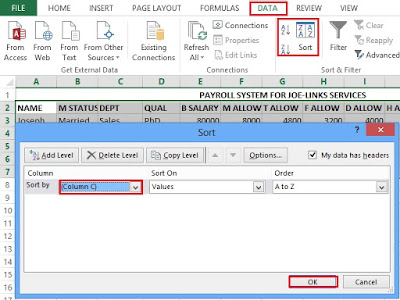One of the attributes of Microsoft Excel that make it unique is its built-in functions that have real life applications both in various fields of study and in business. Some of these built-in functions are:
IF functions
ARITHMETIC functions such as the SUM function, AVERAGE function, etc.
FORECAST function
TREND function
COUNTIF function
SUMIF function
CONCATENATE functions

In this chapter, we will explicitly explain these functions with illustrations and real life applications. I will give you some practical exercises to test your knowledge.

This is the first part (Part 1) of this tutorial chapter (Chapter 3). I will illustrate the business applications of various built-in functions in building result system (grade list), payroll systems and how to apply subtotals to these real life systems.

## IF FUNCTION ANALYSIS

This is a logical function or conditional statement used to evaluate a given condition. It returns the first variable if the condition is true or met, otherwise it returns the second variable. If the condition is false or not met.
Various application software have IF function as one of their built-in functions with slight difference with this Excel IF function syntax.

The syntax is =IF(condition,[value_if_true],[value_if_false]) Enter
Where;
• Condition is the criteria set for excel to use for the evaluation of the records.
• value_if_true is the variable that will be returned if the condition is met.
• value_if_false is the variable that will be returned if the condition is not met. It is most times ignored.

## NESTED IF FUNCTION

This is a special case of IF function whereby an IF function is enclosed in another IF function. It is used when there are more than one conditions to specify. For example, the worksheet in the figure below contains a table for sales record. In the table, there is a Remark column to contain a remark for each sale.If you want Excel to tell you whether you made a profit, loss or you just broke even (I.e. no profit no loss) whenever you enter the item, the quantity sold, the cost price and the selling price, then you will apply a nested IF function in that column.

Place your cursor in cell D2 and type the following nested IF function:
=IF(D2>C2,"profit",IF(D2<C2,"loss",IF(D2=C2,"break even"))) press Enter.
Then use excel fill handle tool to generate a remark for the subsequent cells in the Remark column.

## PRACTICAL ILLUSTRATION/BUSINESS APPLICATIONS OF NESTED IF FUNCTION

I will illustrate the various real-life applications of Arithmetic and Nested IF functions of MS-Excel. Please try to practice these examples on your own.

### STUDENTS RESULT SYSTEM (GRADE LIST)

#### QUESTION:

Given that the grade system of Government Secondary School Owerri is as follows:
0 to 39.9 = F
40 to 49.9 = E
50 to 59.9 = D
60 to 69.9 = C
70 to 79.9 = B
80 to 100 = A
Above 100 = Error

If 50 students participated in the examination of the following courses:
Mathematics, English and Biology, using the above grade system, calculate the following for each student:
1. Total score.
2. Average Score.
3. Grades on the respective subjects.
5. Filter all female students that made an “A” in mathematics.

#### SOLUTION TO THE GRADE LIST SYSTEM QUESTION

You should first create a table like the one contained in the worksheet in the figure below.Result System – Table 1
Type in the following formulas in the appropriate cells and use the hand fill tool to generate the results for the subsequent cells in that column:

1. For the TOTAL SCORE column, place your cursor in cell J3 and type the following formula:
=SUM(D3,F3,H3) press Enter.

2. For the AVERAGE SCORE column, place your cursor in cell I3 and type the following formula:
=J3/3 press Enter. Reduce the number of decimals to two.

3. For the ENGLISH GRADE column, place your cursor in cell E3 and type the following formula:
=IF(D3<40,"F",IF(D3<50,"E",IF(D3<60,"D",IF(D3<70,"C",IF(D3<80,"B",IF(D3<=100,"A",IF(D3>100,"ERROR"))))))) press Enter.

4. For the MATHEMATICS GRADE column, place your cursor in cell G3 and type the following formula:
=IF(F3<40,"F",IF(F3<50,"E",IF(F3<60,"D",IF(F3<70,"C",IF(F3<80,"B",IF(F3<=100,"A",IF(F3>100,"ERROR"))))))) press Enter.

5. For the BIOLOGY GRADE column, place your cursor in cell I3 and type the following formula:
=IF(H3<40,"F",IF(H3<50,"E",IF(H3<60,"D",IF(H3<70,"C",IF(H3<80,"B",IF(H3<=100,"A",IF(H3>100,"ERROR"))))))) press Enter.

6. For the OVERALL GRADE column, place your cursor in cell G3 and type the following formula:
=IF(F3<40,"F",IF(F3<50,"E",IF(F3<60,"D",IF(F3<70,"C",IF(F3<80,"B",IF(F3<=100,"A",IF(F3>100,"ERROR"))))))) press Enter.

The completed Grade List System is shown below.Result System – Table 2
7. Create the three ranges of data. Type “Female” in the Sex column under the criteria range. Type “A” in the Mathematics Grade column under the criteria range.

Use the procedure for performing Advance filter to filter the database based on the specified criteria.

You might need to revisit chapter 2 for the procedure in advance filter.

This is the compilation or list of workers’ information and their payment details in an organization or company. It is a database containing all workers information and their payment details such as basic salary, allowances, gross pay, and net pay.

### DEFINITION OF SOME TERMS USED IN PAYROLL MANAGEMENT

#### 1. PAY SLIP:

This is a detached leaflet or a record from the payroll that contains a worker’s information and payment details.

#### 2. BASIC SALARY:

This is an amount or sum of money a worker is entitled to at the end of the month in a company or organization which is highly influenced by worker’s qualifications and level, and also by the strength of the organization.

#### 3. ALLOWANCE:

This is a fringe benefit that is allocated to every worker in order to encourage workers and enhance their performance in the company or organization.

#### 4.TAX:

This is a compulsory levy that is deducted from a worker’s basic salary at the end of the month in order to generate revenue for the state and federal government.

#### 5.GROSS PAY:

This is the summation of or the total of a worker’s basic salary and all the allowances he is entitled to at the end of every month.

#### 6.NET PAY:

This is Gross pay minus all deductions such as tax and loans. It is the final take-home of a worker at the end of the month.

### PRACTICAL ILLUSTRATION OF A PAYROLL SYSTEM

#### QUESTION:

The manager of JOE-LINKS SERVICES wants to computerize the payroll system of the company. As a spreadsheet expert, design a payroll system to his recommendation and approval to pay his workers on monthly basis such that basic salary is influenced by qualification as follows:

WASSCE = \$20,000
OND = \$25,000
NCE = \$28,000
HND = \$32,000
BSc = \$40,000
MSc = \$50,000
PhD = \$80,000 respectively.

Marital allowance would be 8% and 10% for single and married workers respectively. Transport, Feeding, Dressing and Housing allowances would be 6%, 4%, 5%, and 8% of their basic salaries respectively.

Compute a tax deduction such that:
WASSCE = 5%, OND = 6%, NCE = 8%, HND = 10%, BSc = 12%, MSc = 15% and PhD = 20% of their basic salaries respectively.

Calculate the Gross pay and Net pay for each worker.

Setup an advanced query or filter to filter all the married workers with qualification of BSc.

### SOLUTION TO THE PAYROLL SYSTEM

You should first create a table like the one contained in the spreadsheet in the figure below:
Type in the following formulas in the appropriate cells and use the hand fill tool to generate the results for the subsequent cells in that column:

1. For the BASIC SALARY column, place your cursor in cell D3 and type the following formula:
=IF(C4="WASSCE",20000,IF(C4="OND",25000,IF(C4="NCE",28000,IF(C4="HND",32000,IF(C4="BSc",40000,IF(C4="MSc",50000,IF(C4="PhD",80000,0))))))) press Enter.

2. For the MARITAL ALLOWANCE column, place your cursor in cell E3 and type the following formula:
=IF(B3="Single",D3*8%,IF(B3="Married",D3*10%,0)) press Enter.

3. For the TRANSPORT ALLOWANCE column, place your cursor in cell F3 and type the following formula:
=D3*6% press Enter.

4. For the FEEDING ALLOWANCE column, place your cursor in cell G3 and type the following formula:
=D3*4% press Enter.

5. For the DRESSING ALLOWANCE column, place your cursor in cell H3 and type the following formula:
=D3*5% press Enter.

6. For the HOUSING ALLOWANCE column, place your cursor in cell E3 and type the following formula:
=D3*8% press Enter.

7. For the TAX column, place your cursor in cell J3 and type the following formula:
=IF(C3="WASSCE",D3*5%,IF(C3="OND",D3*6%,IF(C3="NCE",D3*8%,IF(C3="HND",D3*10%,IF(C3="BSc",D3*12%,IF(C3="MSc",D3*15%,IF(C3="PhD",D3*20%,0))))))) press Enter.

8. For the GROSS PAY column, place your cursor in cell K3 and type the following formula:
=SUM(D3:I3) press Enter.

9. For the NET PAY column, place your cursor in cell L3 and type the following formula:
=K3-J3 press Enter.
The completed Payroll System is shown below.Payroll System – Table 2
10. Create the three ranges of data. Type “Married” in the M Status column under the criteria range. Type “BSc” in the Qual column under the criteria range.

Use the procedure for performing Advance filter to filter the database based on the specified criteria.
You might need to revisit chapter 2 - part 1  for the procedure in advance filter.

## SUBTOTAL AND HOW TO APPLY IT IN AN EXCEL WORKSHEET

Subtotal is an Excel tool used to calculate the total value or amount spent in a particular group of records in a database. It is mainly used in Payroll and Budget Control Systems to calculate the total amount spent in each department of a company.

### To Apply Subtotal to a Database in an Excel Worksheet:

1. Prepare and activate the worksheet containing the database you wish to use, for example, a payroll database.

2. Sort the records using the Department column as your sorting field by clicking Sort located in the Sort & Filter group in the Data tab. Select the sort column from the Sort By drop down menu and click OK as shown below.Subtotal sort
3. Click on Subtotal located in the Outline group of Data tab. The subtotal dialogue box appears.

4. Specify the changing field from the AT EACH CHANGE IN drop down menu which in this case should be the Department column.

5. Specify the ADD SUBTOTAL TO field. In the case of a payroll system, specify either the Gross Pay or the Net Pay column depending on your intention.

6. Put a check the Replace current subtotals and Page break between groups boxes and click OK as shown below.Subtotal dialogue
Excel does the calculation for you and returns the values in a row below the last record in each group as shown below.

## SOME SAMPLE QUESTIONS UNDER GRADE LIST SYSTEM AND PAYROLL SYSTEM

1. Government Secondary School Owerri wants to computerize her students result in the following subjects:
English, Mathematics, Chemistry, Physics, Biology, Economics, Literature and Government.

a.) As a Spreadsheet Manager, design a result System to compute the Total Score and Average Score.

b.) Using IF function, generate the grade letters for each subject and the overall grade using the following grade Scheme:
0 – 39.9 = Fail
40 – 49.9 = Pass
50 – 59.9 = Credit
60 – 69.9 = Upper Credit
70 – 100 = Distinction

c.) Generate 30 records and Set up an advance filter to filter Science Students from Imo State.

2. a.) Zenith Bank PLC wants to computerize her worker’s payroll system to compute the Basic Salary based on the following qualifications:

SSCE = \$1200
NCE = \$1300
HND = \$1500
BSc = \$1800
MSc = \$2200
PhD = \$2800

b.) Compute 8%, 12%, 7.5%, 6% and 5% of the Basic Salary as Housing, Transport, Medical, Feeding and Dressing allowances.
c.) Tax deduction should be calculated as follows:
SSCE = 1.5%
NCE = 2%
HND = 2.5%
BSc = 3%
MSc = 3.5%
PhD = 4%

d.) Calculate the Gross and Net Pay.

e.) Set up a subtotal to calculate the total amount spent in each department.

This is the end of this part.
Now go over to the next part of this tutorial chapter:

Don’t forget to subscribe to get our latest tutorial  post delivered to your email.

## Recommended Entrepreneurship BooksThe Passionate Entrepreneur’s Strategies: Learn how to discover business ideas that will be successful, grow multiple online income streams, make money from home, attract new and retain customers, applications of SWOT analysis in any business, skills that will help you succeed as an entrepreneur, self-discipline, etc. Lucrative Business Ideas Series Book 1. By Buzzer Joseph. Read more about the book.How to Make Money from Blogging and Affiliate Marketing: Learn the Step by Step Guide of Creating, Growing and Optimizing your WordPress Blog from Scratch for Search Engines (SEO) and How to Make Money through Google AdSense, Affiliate Marketing and Other Strategies for Earning Passive Income Online. Lucrative Business Ideas Series Book 2. By Buzzer Joseph. Read more about the book.How to Make Money Self-Publishing Kindle Books for Beginners: Learn the Step by Step Guide to Self-Publish a Best Selling Book on Amazon KDP, How to Design Book Covers with Adobe Photoshop, Format, Edit and Proofread your Book Manuscript with Microsoft Word, Promote your Book on Various Online Platforms and Earn Monthly Passive Income Online. Lucrative Business Ideas Series Book 3. By Buzzer Joseph. Read more about the book.Complete Solar PV System Installation Guide: DIY guide on how to install grid-connected and off-grid solar PV systems, how to build solar-powered electric cars, convert conventional cars to electric cars, online and offline ways to make money from solar energy sector (including stocks), electrical wiring and protection system installation guides, plus free resources for skill acquisition and personal development. Lucrative Business Ideas Series Book 4. By Buzzer Joseph. Read more about the book.How to Make Money from Crypto Trading and Investing for Beginners: Step by step guide with screenshots on the basics of blockchain technology, bitcoin and altcoins, crypto security tips, cryptocurrency market analysis (FA and TA), long term and short term trading, how to calculate your profits in excel, legit ways to make money with cryptocurrencies, DeFi yield farming and staking, how to use any centralized and decentralized exchange platform and more, plus crypto investment bonus! Lucrative Business Ideas Series Book 5. By Buzzer Joseph. Read more about the book.